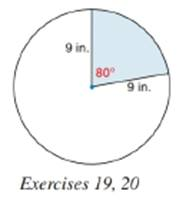Chapter 8.5, Problem 19E### Elementary Geometry for College St...

6th Edition
Daniel C. Alexander + 1 other
ISBN: 9781285195698

#### Solutions

Chapter
Section### Elementary Geometry for College St...

6th Edition
Daniel C. Alexander + 1 other
ISBN: 9781285195698
Textbook Problem
1 views

# Find the approximate perimeter of the sector shown. Answer to the nearest hundredth of an inch.To determine

To find:

The approximate perimeter of the sector.

Explanation

Definition:

Arc Measure:

In a circle, the degree measure of an arc is equal to the measure of central angle that intercepts the arc.

Formula:

Perimeter of sector:

If r is the radius of circle,

Psector=r+r+lintercepted arc=2r+lintercepted arc

Thus, Psector=2r+lintercepted arc

Length of an arc:

In a circle whose circumference is C, the length l of an arc whose degree measure is m is given by:

l=m360×C

We know that, circumference of a circle is given by 2πr where r is the radius of circle.

Hence, length l of an arc =m360×2πr

Calculation:

Perimeter of sector:

From the diagram it is clear that radius of the circle is 9 in.

The measure of central angle is given as 800.

As per the definition of measure of an arc, the degree measure of an arc is equal to the central angle intercepted by it.

Thus, the degree measure of arc =800

### Still sussing out bartleby?

Check out a sample textbook solution.

See a sample solution

#### The Solution to Your Study Problems

Bartleby provides explanations to thousands of textbook problems written by our experts, many with advanced degrees!

Get Started

#### List all of the subsets of {a, b, c, d}.

Mathematical Excursions (MindTap Course List)

#### 74. Find S' if S =

Mathematical Applications for the Management, Life, and Social Sciences

#### True or False: is a convergent series.

Study Guide for Stewart's Multivariable Calculus, 8th

#### Given the Taylor Series , a Taylor series for ex/2 is:

Study Guide for Stewart's Single Variable Calculus: Early Transcendentals, 8th

#### Describe how replication protects against fraud being committed in research.

Research Methods for the Behavioral Sciences (MindTap Course List)